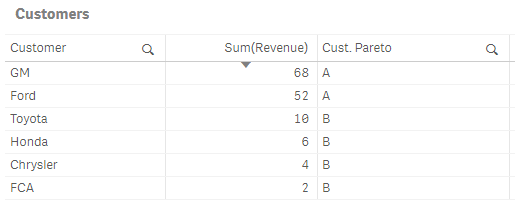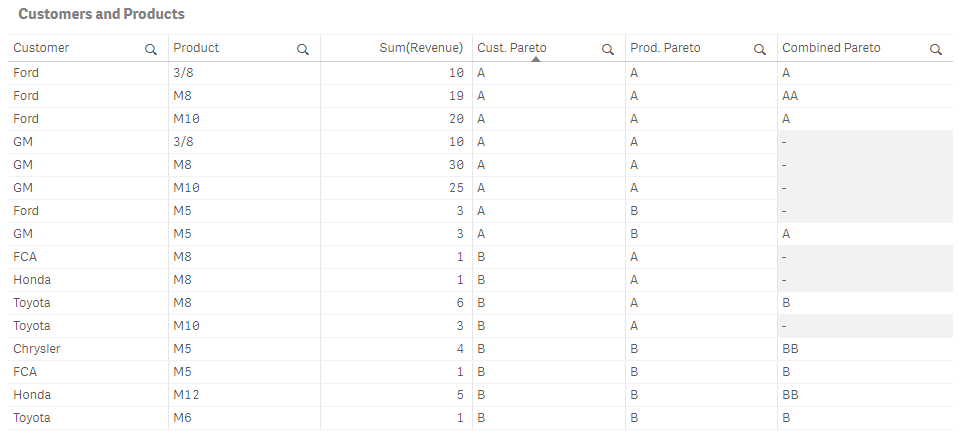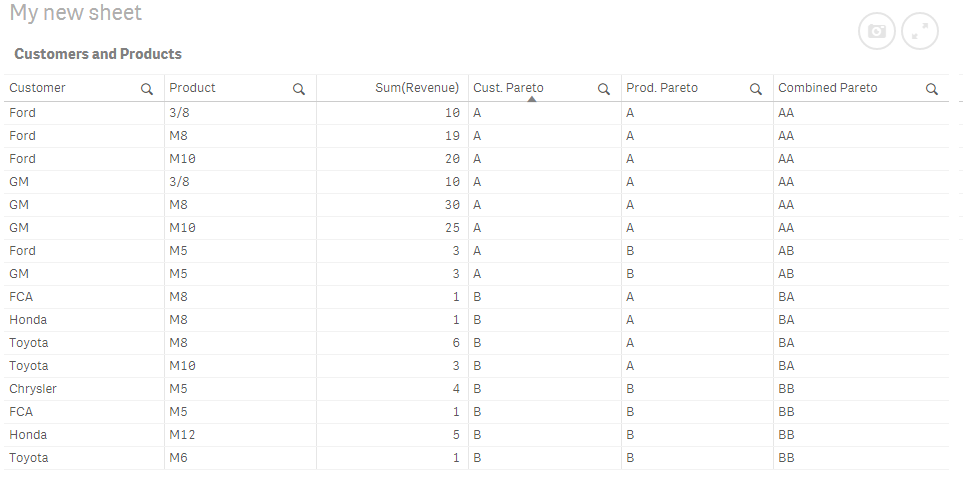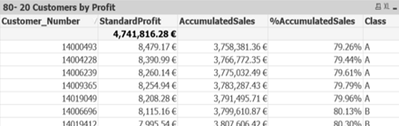# App Development

Announcements
April 22, 2PM EST: Learn about GeoOperations in Qlik Sense SaaS READ MORE
cancel
Showing results for
Did you mean:Creator II

## Combined Pareto class dimension

I am working on a Pareto dashboard.  My work so far is based on this article:

https://community.qlik.com/t5/Qlik-Design-Blog/Recipe-for-a-Pareto-Analysis-Revisited/ba-p/1473684

My customer Pareto dimension works fine:

``````= Aggr(
If(Rangesum(Above(Sum({1} Revenue), 1, RowNo())) /
Sum({1} TOTAL Revenue) < 0.8, 'A', 'B'),
(Customer, (=Sum({1} Revenue), Desc))
)``````My product Pareto dimension also works fine:

``````= Aggr(
If(Rangesum(Above(Sum({1} Revenue), 1, RowNo())) /
Sum({1} TOTAL Revenue) < 0.8, 'A', 'B'),
(Product, (=Sum({1} Revenue), Desc))
)``````Now, I am trying to figure out how to make a combined class dimension.  In other words, if a straight table row contains an A customer and an A product, the combined class should be AA.

Naively, I tried this for the dimension (just concatenating the other two dimensions):

``````= Aggr(
If(Rangesum(Above(Sum({1} Revenue), 1, RowNo())) /
Sum({1} TOTAL Revenue) < 0.8, 'A', 'B'),
(Customer, (=Sum({1} Revenue), Desc))
)
& Aggr(
If(Rangesum(Above(Sum({1} Revenue), 1, RowNo())) /
Sum({1} TOTAL Revenue) < 0.8, 'A', 'B'),
(Product, (=Sum({1} Revenue), Desc))
)``````

As you can see in the picture below, it doesn't work right.  I guess it makes sense that it doesn't work since I'm just concatenating rows from two virtual tables of different lengths with no meaningful relationship to one another.  I need to "join" them somehow.Note that I cannot do this work in SQL or in the load script because I will eventually replace the above set analysis ({1}) with something more complex that facilitates limited interactivity (dynamically updating Pareto classes based on date selections).

I attached the .qvf file, and here's just the data:

``````SalesData:
Customer|Product|Revenue
Ford|M8|4
Ford|M8|15
Ford|M5|3
Ford|M10|20
Ford|3/8|10
GM|M5|3
GM|M10|25
GM|M8|30
GM|3/8|10
Toyota|M8|6
Toyota|M10|3
Toyota|M6|1
Honda|M12|5
Honda|M8|1
FCA|M5|1
FCA|M8|1
Chrysler|M5|4
] (delimiter is '|');``````

Labels (3)

• ### AGGR()

1 Solution

Accepted SolutionsMVP

May be try adding NODISTINCT within the Aggr() functions

``````=
Aggr(NODISTINCT
If(Rangesum(Above(Sum({1} Revenue) / Sum({1} TOTAL Revenue), 1, RowNo())) < 0.8, 'A', 'B'),
(Customer, (=Sum({1} Revenue), Desc))
) &
Aggr(NODISTINCT
If(Rangesum(Above(Sum({1} Revenue) / Sum({1} TOTAL Revenue), 1, RowNo())) < 0.8, 'A', 'B'),
(Product, (=Sum({1} Revenue), Desc))
)``````3 RepliesMVP

May be try adding NODISTINCT within the Aggr() functions

``````=
Aggr(NODISTINCT
If(Rangesum(Above(Sum({1} Revenue) / Sum({1} TOTAL Revenue), 1, RowNo())) < 0.8, 'A', 'B'),
(Customer, (=Sum({1} Revenue), Desc))
) &
Aggr(NODISTINCT
If(Rangesum(Above(Sum({1} Revenue) / Sum({1} TOTAL Revenue), 1, RowNo())) < 0.8, 'A', 'B'),
(Product, (=Sum({1} Revenue), Desc))
)``````Creator II
Author

Awesome, thanks!

For others who find this solution and wonder what NODISTINCT does, I found this post with an example similar to my situation helpful:
https://community.qlik.com/t5/QlikView-App-Development/What-NODISTINCT-parameter-does-in-AGGR-functi...Partner

Hello All,

I am new to Qlikview and I am working on 80-20(Pareto) Analysis and Quadrant map in QlikView.

I replicated the below link which explains it very well and my 80-20 chart for Customers and Products works fine

https://community.qlik.com/t5/Qlik-Design-Blog/Recipe-for-a-Pareto-Analysis/ba-p/1468497Now, I am trying to match Customer_Number from Straight Table 1 and Product_Line from Straight Table 2 based on its Class and create four Quadrants that shows number of Customers & products from Class AA, Class AB, Class BA, and Class BB

I followed the below link to do that but unfortunately my Qlikview version does not support the expression.

https://community.qlik.com/t5/Qlik-Design-Blog/Recipe-for-a-Pareto-Analysis-Revisited/ba-p/1473684

The expressions that are not working in my Qlikview version 11 are

Customers:

=Aggr(If(Rangesum(Above(Sum({1} Standard_Profit)/Sum({1} total Standard_Profit),1,RowNo()))<=0.8, 'A','B'),
(Customer_Number,(=Sum({1}Standard_Profit),Desc)))

Products:

=Aggr(If(Rangesum(Above(Sum({1} Standard_Profit)/Sum({1} total Standard_Profit),1,RowNo()))<=0.8, 'A','B'),
(Product_Line,(=,(=Sum({1}Standard_Profit),Desc)))

To Join the classes between Customers and Products:

=Aggr(NODISTINCT If(Rangesum(Above(Sum({1} Standard_Profit)/Sum({1} total Standard_Profit),1,RowNo()))<=0.8, 'A','B'),
(Customer_Number,(=Sum({1}Standard_Profit),Desc)))

&

=Aggr(NODISTINCT If(Rangesum(Above(Sum({1} Standard_Profit)/Sum({1} total Standard_Profit),1,RowNo()))<=0.8, 'A','B'),
(Product_Line,(=,(=Sum({1}Standard_Profit),Desc)))

Now, I must group the classes AA, AB, BA, BB and create four lists that shows -

Quadrant 1: List of Customer Numbers with class 'A' from Straight Table 1 that has Product Line Class 'A' from straight table 2. Eg., Customer_Numbers in class A that has Product_Line in class A

Quadrant 2: List of Customer Number with class 'A' from Straight Table 1 that has Product Line Class 'B' from straight table 2. Eg., Customer_Numbers in class A that has Product_Line in class B

Quadrant 3: List of Customer Number with class 'B' from Straight Table 1 that has Product Line Class 'A' from straight table 2 Eg., Customer_Numbers in class B that has Product_Line in class A

Quadrant 4: List of Customer Number with class 'B' from Straight Table 1 that has Product Line Class 'B' from straight table 2. Eg., Customer_Numbers in class B that has Product_Line in class B

Can anyone please help me? It is very important for my project and a little urgent one. I would highly appreciate your help. I have attached my file for your reference Thanks a lot, in advance.Tags
Community Browser